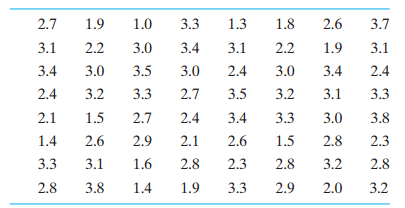### Create an Account

Home / Questions / For the grouped scores in Problem 15 determine a P80 bP20 education Problem 15 A small ...

# For the grouped scores in Problem 15 determine a P80 bP20 education Problem 15 A small eastern college uses the grading system of 040with 40 being the highest possible grade The scores

For the grouped scores in Problem 15, determine

a. P80

b.P20 education

Problem 15

A small eastern college uses the grading system of 0–4.0, with 4.0 being the highest possible grade. The scores shown here are the grade point averages of the students currently enrolled as psychology majors at the college.a. Construct a frequency distribution of grouped scores with approximately 10 intervals.

b. Construct a histogram of the frequency distribution constructed in part a.

c. Is the distribution skewed or symmetrical? If skewed, is it skewed positively or negatively?

d. Construct a stem and leaf diagram with the last digit being a leaf and the first digit a stem. Repeat stem values five times.

e. Which diagram do you like better, the histogram of part b or the stem and leaf diagram of part d? Explain. Education

Apr 01 2020 View more View LessSubscribe To Get Solution